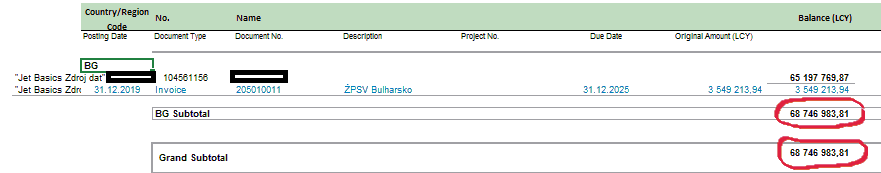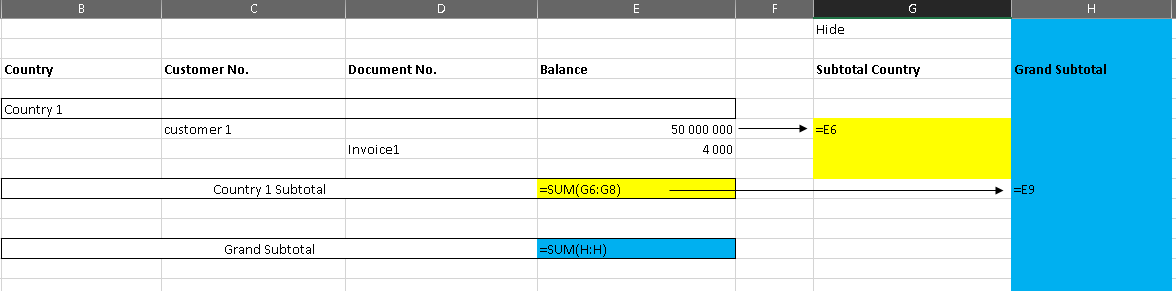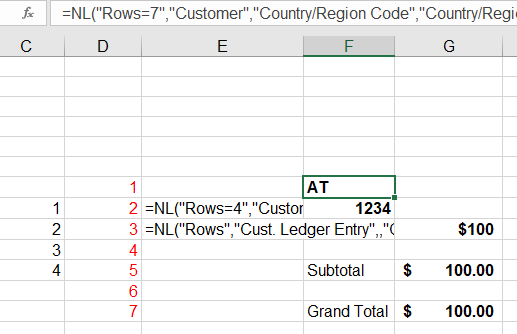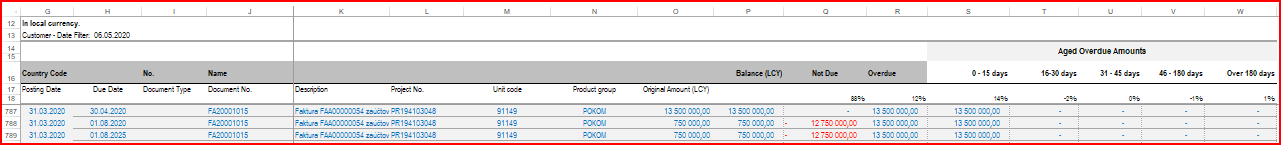`Hi everyone,I am trying to create AR report. I need to have a report that showsme the country, customer and his outstanding invoices. And thenI need to make a subtotal of numbers according to country and at the same time the total number. But the subtotal according to countryis not working. I am convinced that it is caused by formula in bold mentioned below(Rows=5, rows=3).cell F20  =NL("Rows=5";"18 Customer";"35 Country/Region Code")cell E21 =NL("Rows=3";"18 Customer";;"1 No.";\$H\$6;"+2 Name";"*";"35 Country/Region Code";F20;"61 Net Change (LCY)";"<>0";"55 Date Filter";\$N\$3)cell E22 =NL("Rows";"21 Cust. Ledger Entry";;"3 Customer No.";\$G21;"4 Posting Date";\$N\$3;"14 Remaining Amount";"<>0";"76 Date Filter";\$N\$3)`

Please, any help is appreciated. Thanks a lot.Petra

•Florian Rauber

Hello Petra,

you can use an easy trick to sum up the right balances. You have to link the subtotal of you customers of each country in an other cell (on the right side for example) and sum up this column. Thats the easiest way.

Here is an example:The yellow SUM formula should be expanded when you start your report and should sum up all the subtotals of your customers. The sum up of your Grand subtotal should be the same.

Greetings

Florian

Edited by Florian Rauber
•Bryan Robinson

Since we can't see how you are getting your subtotal and grand total, it is a bit hard to provide a true answer but when doing grouping reports with subtotals, it is best to use Excel's SUBTOTAL function as it will ignore any other SUBTOTAL functions within the range.  https://support.office.com/en-us/article/subtotal-function-7b027003-f060-4ade-9040-e478765b9939

•Amy W

Two ideas:

1.  Instead of Rows = 5 and Rows = 3, try using Rows = 7 and Rows=4.

2.  Instead of using the SUM function, try using SUBTOTAL(9,•Petra Janásová

Dear all,

after a long time spending above the mentioned issue I have finally figured out the subtotal for each country. Despite all that I am very grateful for you help. I appreciate it a lot.

Many thanks. Petra ;-)

Now, I am solving different issue regarding outstanding amounts. Let us assume that our customer´s invoice is divided into 3 amounts. Each of them is payable at a different day. I am talking about a current receivable, short-term and long-term retention amounts.

Shortly: In the picture below we can see the invoice no. FA20001015 - the whole amount is 15k. According mentioned above the amount is divided into amounts 13,5k, and twice 750t.

But what the problem is that in the cells Q788(789) and R788(789) we can see the diference between the amounts to see the wright figures in comun Balance (750t). So, the balance would be good but when I take the total figure which should show me the "Not Due" and "Overdue" - it is not wright. Pls, is there any way how not to show these figures (-12,75k and 13,5k) and it would show me only 750t?

column P=NF(\$F22;"16 Remaining Amt. (LCY)";"76 Date Filter";\$Q\$3)

column Q=P22-R22

column R=IF(\$F22<>"";NL("Sum";"21 Cust. Ledger Entry";"16 Remaining Amt. (LCY)";"6 Document No.";\$J22;"37 Due Date";\$Q\$3;"76 Date Filter";\$Q\$3);0)